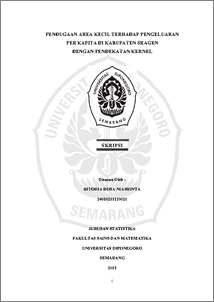# PENDUGAAN AREA KECIL TERHADAP PENGELUARAN PER KAPITA DI KABUPATEN SRAGEN DENGAN PENDEKATAN KERNEL

NIASHINTA, BITORIA ROSA (2016) PENDUGAAN AREA KECIL TERHADAP PENGELUARAN PER KAPITA DI KABUPATEN SRAGEN DENGAN PENDEKATAN KERNEL. Undergraduate thesis, FSM Universitas Diponegoro.Preview
PDF
3424Kb

## Abstract

Data of Social Survey and Economic National is a relatively small sample of data, so that data is called small area. Estimation of parameter in small area can be done in two ways, there are direct estimation and indirect estimation. Direct estimation is unbias estimation but give a high variance because from small sample of data. The technique that use to increase efectivity of sample size is indirect estimation or called Small Area Estimation (SAE). SAE is done by adding auxiliary variable. on estimating parameter. Assumed that auxiliary variable has a linear correlation with the direct estimation. If that assumption is incomplete, use an nonparametric approaching. This research is using Kernel Gaussian approaching to build a correlation between direct estimation which expenditure per capita and auxiliary variable which population density. Evaluation of estimation result is done by comparing the value of direct estimation variance with the value of indirect estimation variance using Kernel Gaussian approaching. The result of parameter estimation which approached by SAE is the best estimation, because it produce the small value of variance that is 5,31275, while the value of direct estimator variance is 6,380522. Keywords: Direct Estimation, Small Area Estimation (SAE), Kernel Gaussian.

Item Type: Thesis (Undergraduate) H Social Sciences > HA Statistics Faculty of Science and Mathematics > Department of Statistics 49826 Mr Hasbi Yasin 23 Aug 2016 09:23 23 Aug 2016 09:23

Repository Staff Only: item control page